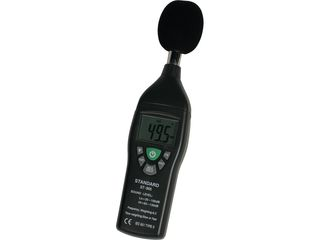# Jargon buster: DecibelThe decibel (dB) is a unit of measurement used for all kinds of things, not just audio. It is a relative measurement, meaning that it expresses a difference in magnitude.

When setting the fader in a mixer to make a sound louder or quieter, for instance, we use dB values to express the change in level applied to the signal . An increase of 6dB equates to a doubling of the amplitude of the sound - if you looked at the waveform before and after, it would be twice as tall (double the amplitude) after the 6dB boost.

To then halve the level of a signal, you would attenuate by 6dB, which is expressed as a negative value: -6dB. Add 6dB and -6dB together and you get 0dB, ie, no change.

One advantage of using decibels rather than ratios such as 2 or1/2 is that we simply add decibel values to find out the final result, rather than multiplying as we would with ratios.

When talking about digital signals in our DAWs, we also use decibels to express the level relative to the absolute maximum that an audio interface can reproduce before clipping: 0dBFS (dB Full Scale). When we refer to, say, a signal "peaking at -6dB", we really mean -6dBFS, given that dB is a relative measurement.

The dynamic range of a system is the difference between 0dBFS and the average level (aka RMS) of the noise floor. You may also have heard dB used to describe the level of real-world sounds, which is misleading - dBSPL is the real unit here. The reference point is an SPL that's agreed to be just at the threshold of our hearing sensitivity, so any sounds that are less than 0dBSPL should be inaudible to us.

One final point of interest: although 6dB represents the doubling of a signal's amplitude, we do not necessarily perceive this as 'twice as loud'. An increase of 10dB is supposedly twice as loud, although this is a psychological phenomena, and so ultimately subjective.